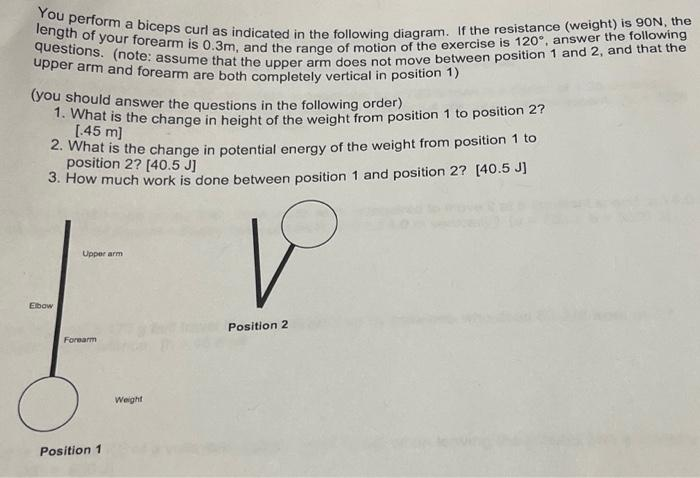Home / Expert Answers / Physics / you-perform-a-biceps-curl-as-indicated-in-the-following-diagram-if-the-resistance-weight-is-90-pa244

# (Solved): You perform a biceps curl as indicated in the following diagram. If the resistance (weight) is 90 ...You perform a biceps curl as indicated in the following diagram. If the resistance (weight) is , the length of your forearm is , and the range of motion of the exercise is , answer the following questions. (note: assume that the upper arm does not move between position 1 and 2 , and that the upper arm and forearm are both completely vertical in position 1) (you should answer the questions in the following order) 1. What is the change in height of the weight from position 1 to position 2 ? 2. What is the change in potential energy of the weight from position 1 to position 3. How much work is done between position 1 and position

We have an Answer from Expert